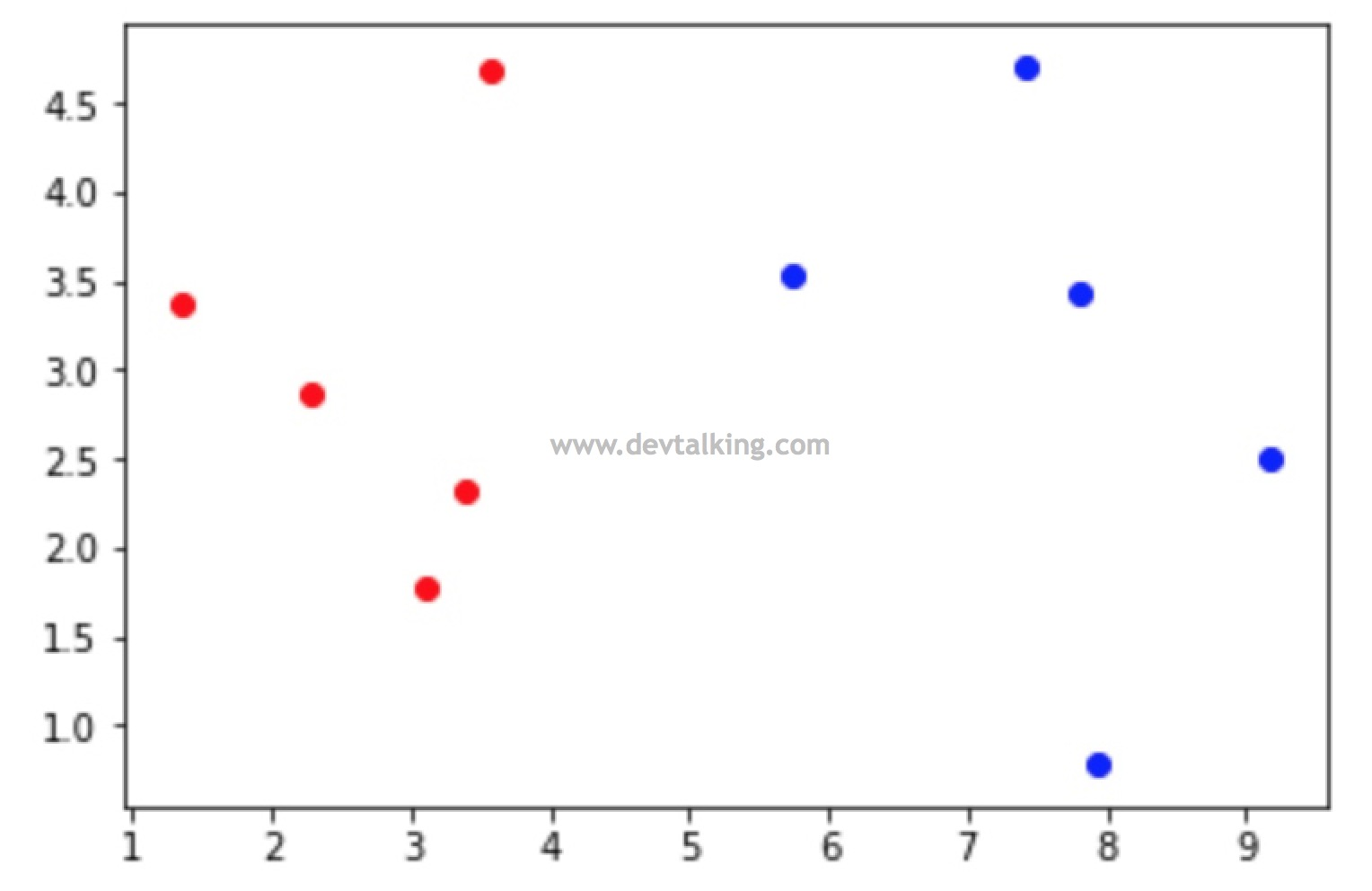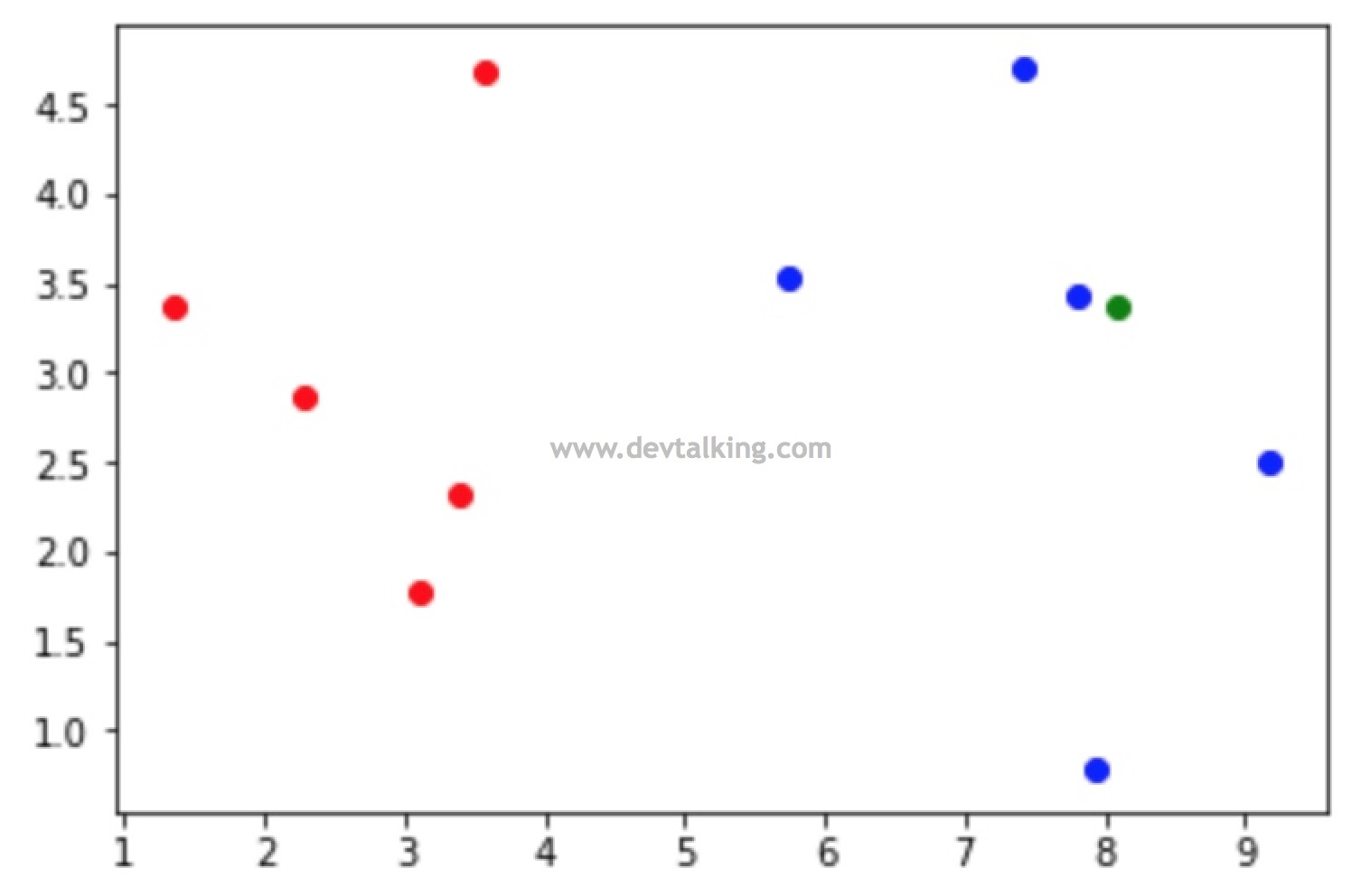NumPy

NumPy是Python中的一个类库，它支持高阶维度数组（矩阵）的创建及各种操作、运算，是我们在机器学习中经常会使用的一个类库。这一节再看一些NumPy的矩阵用法。

numpy.random

NumPy也提供了生成随机数和随机元素数组的方法，我们来看一下：

指定均值和标准差生成随机数数组或矩阵

$$\overline X=\frac{\sum_{i=1}^nX_i}n$$

$$S^2 = \frac{\sum_{i=1}^n(X_i-\overline X)^2} n$$

$$S = \sqrt {\frac{\sum_{i=1}^n(X_i-\overline X)^2} n }$$

NumPy也提供了指定均值和标准差生成随机数的方法，我们来看一下：

切片

Python中，有一个获取数组片段非常方便的方法，叫做切片，numpy.array中同样支持切片，我们来看一下：

改变数组维度

NumPy也提供了修改数组维度的方法，我们来看看：

数组合并操作

NumPy也提供两个数组合并的操作：

矩阵运算

NumPy中提供了完整的矩阵的运算，我们从加减法来看一下：

聚合操作

NumPy中有很多对数组的聚合操作方法，我们先来看看一维数组：

索引和排序的相关操作

NumPy提供了一系列对数组索引操作的方法，我们来看一下：

Matplotlib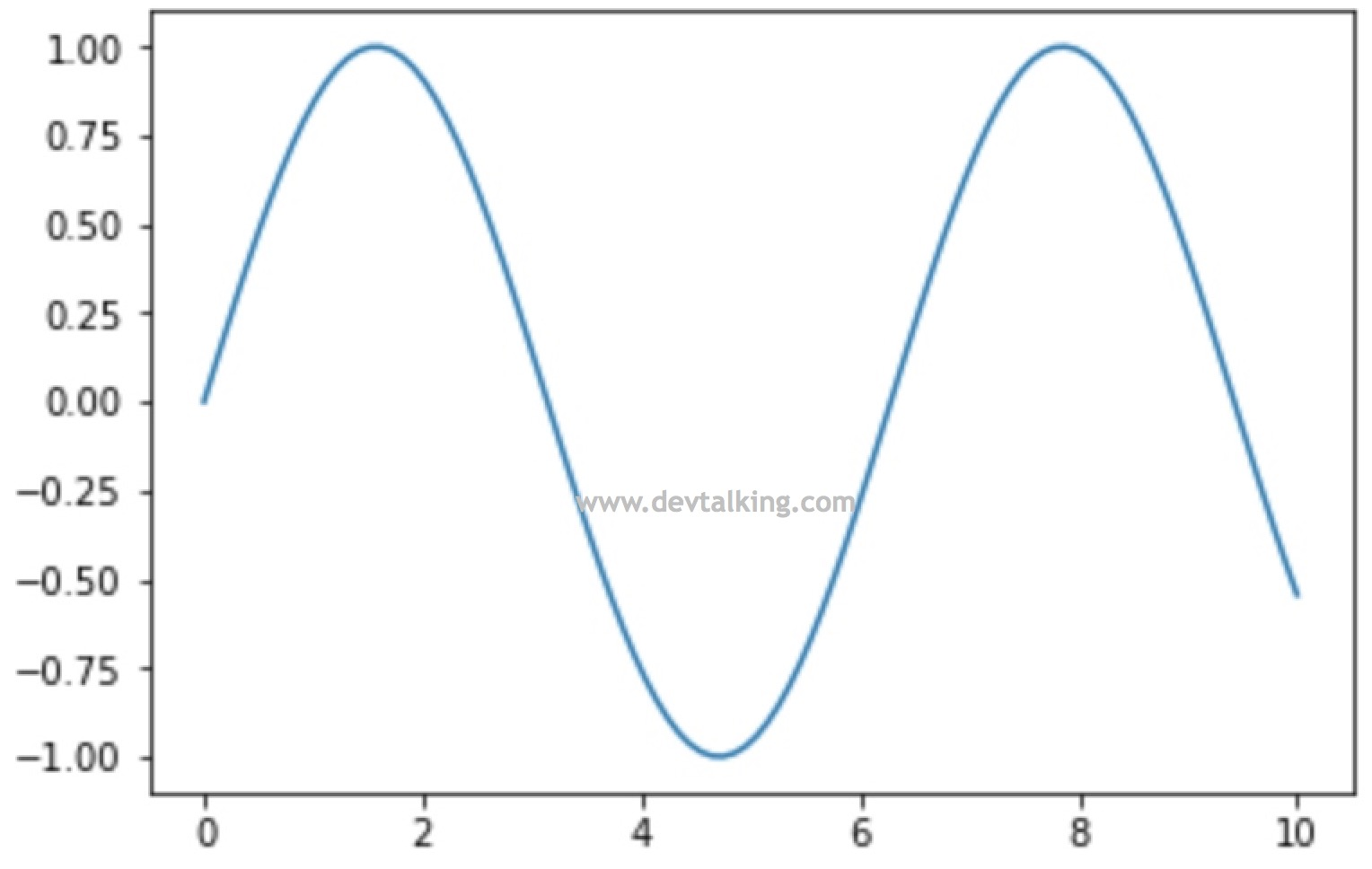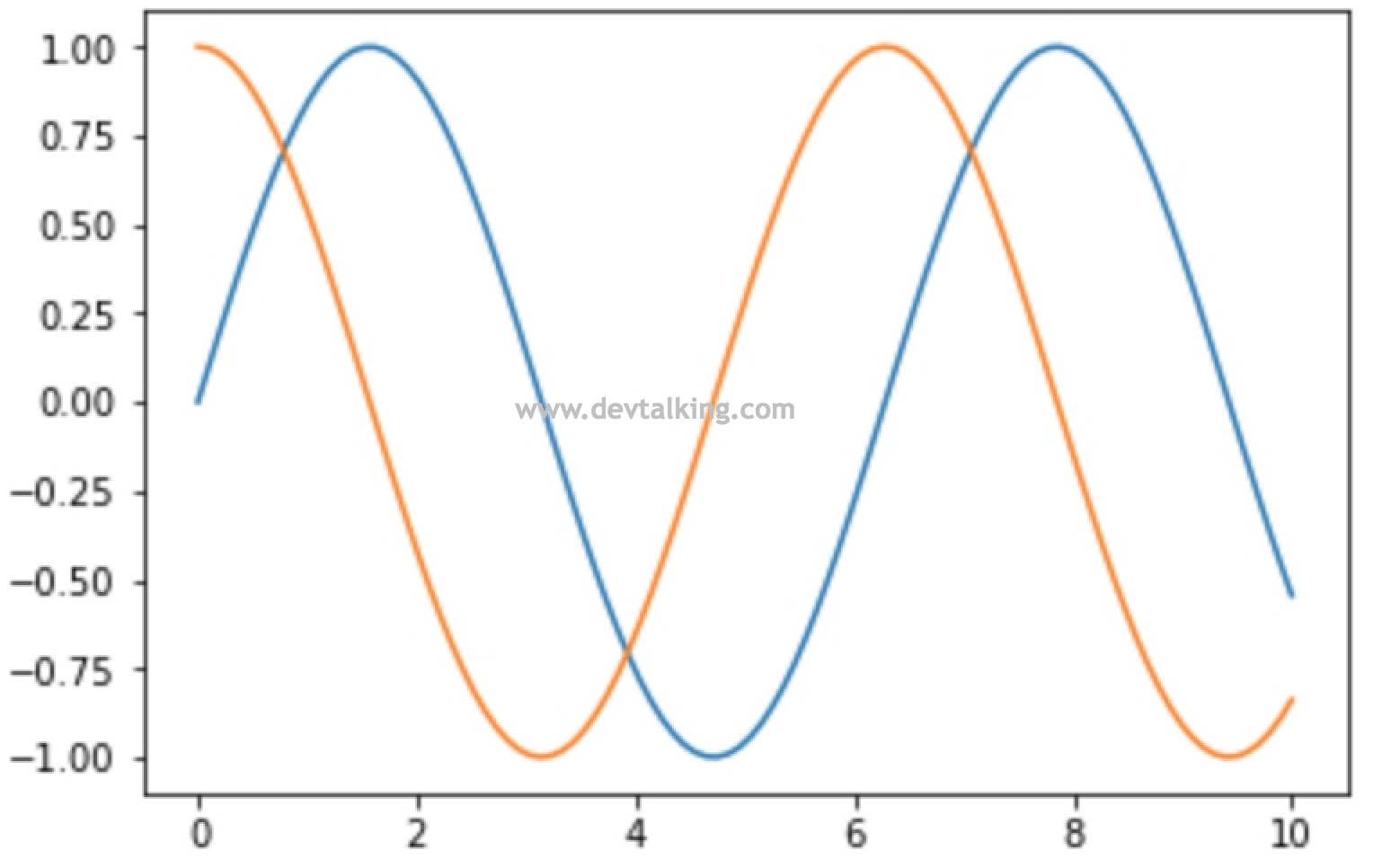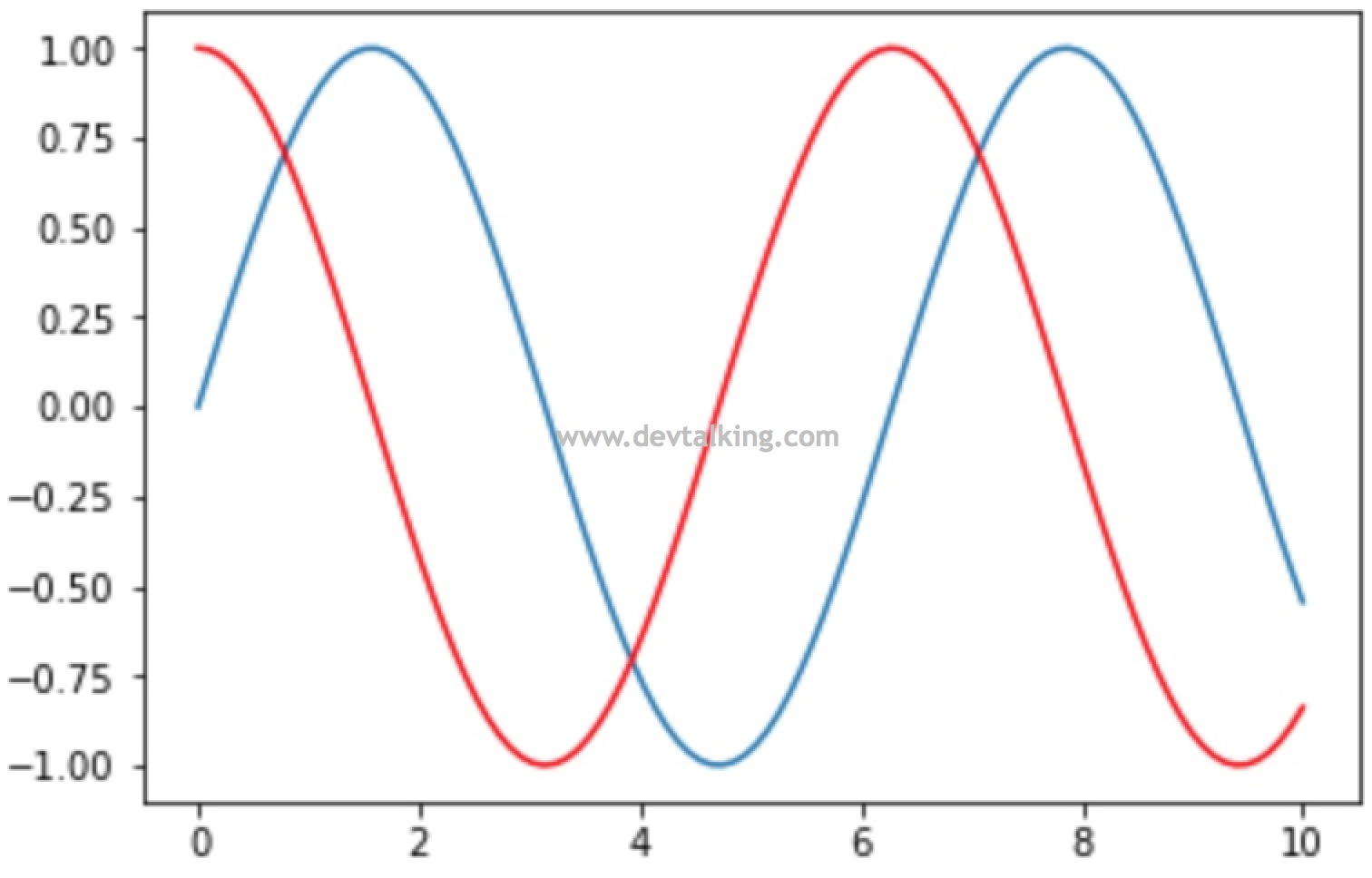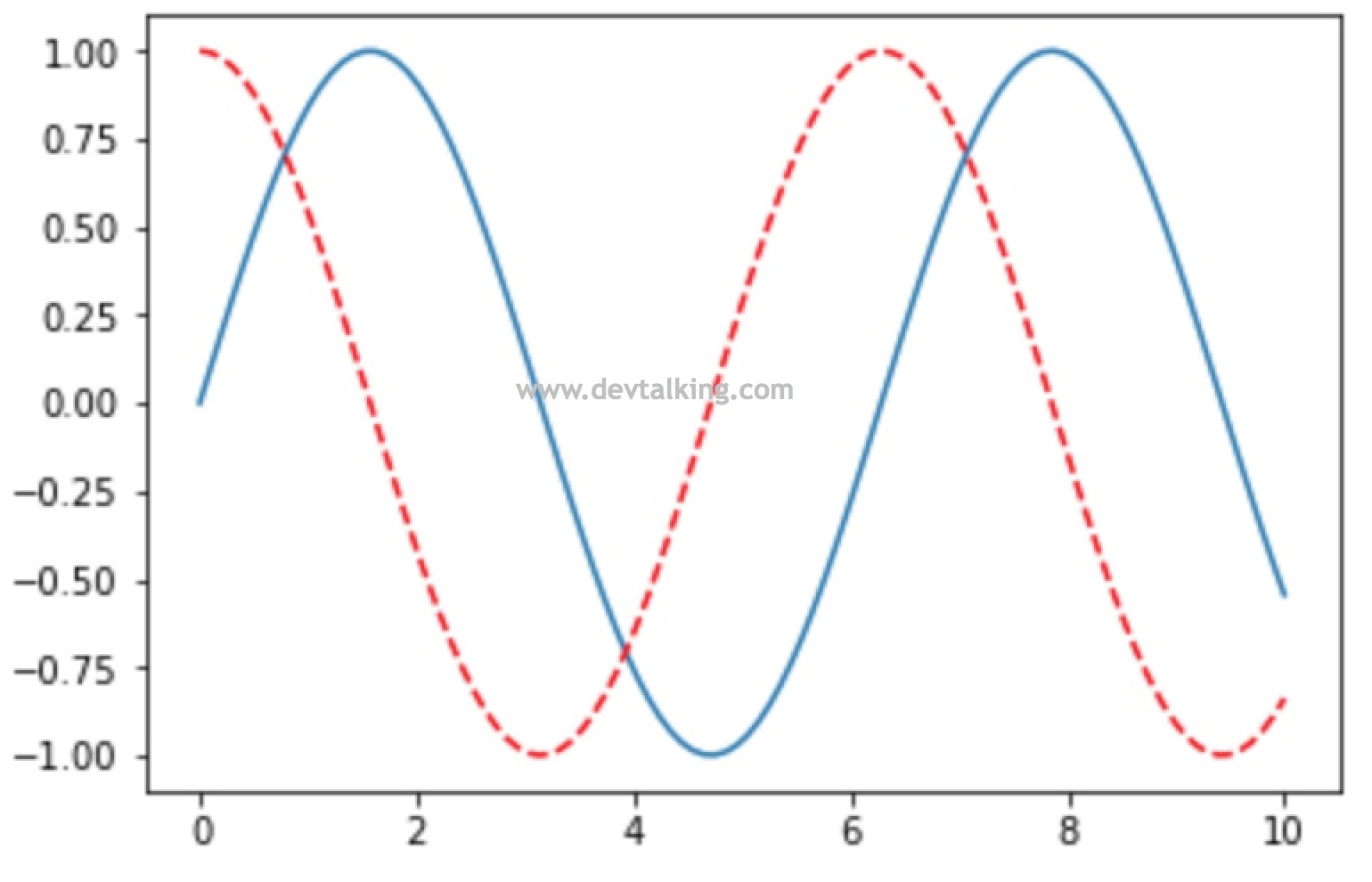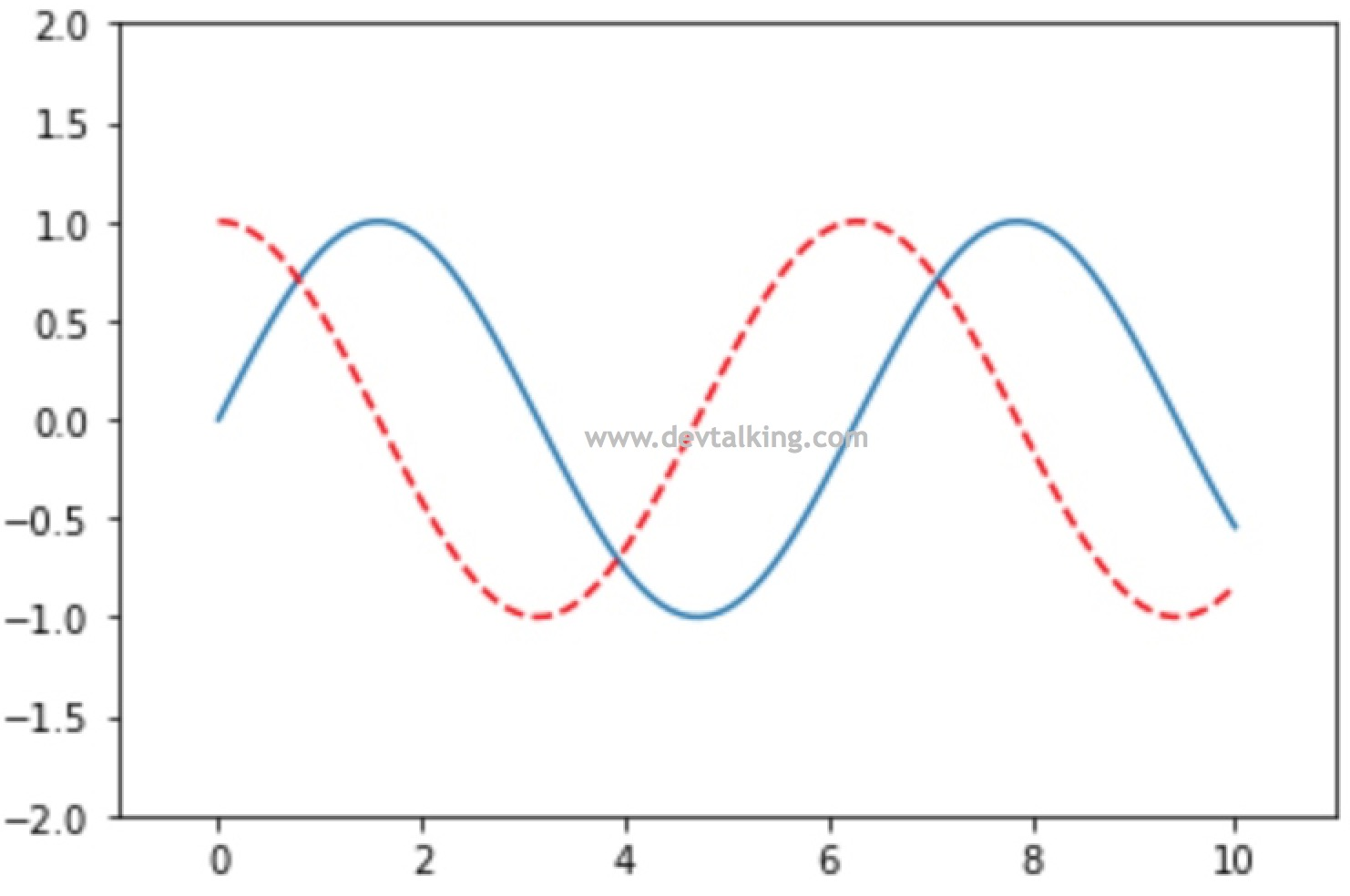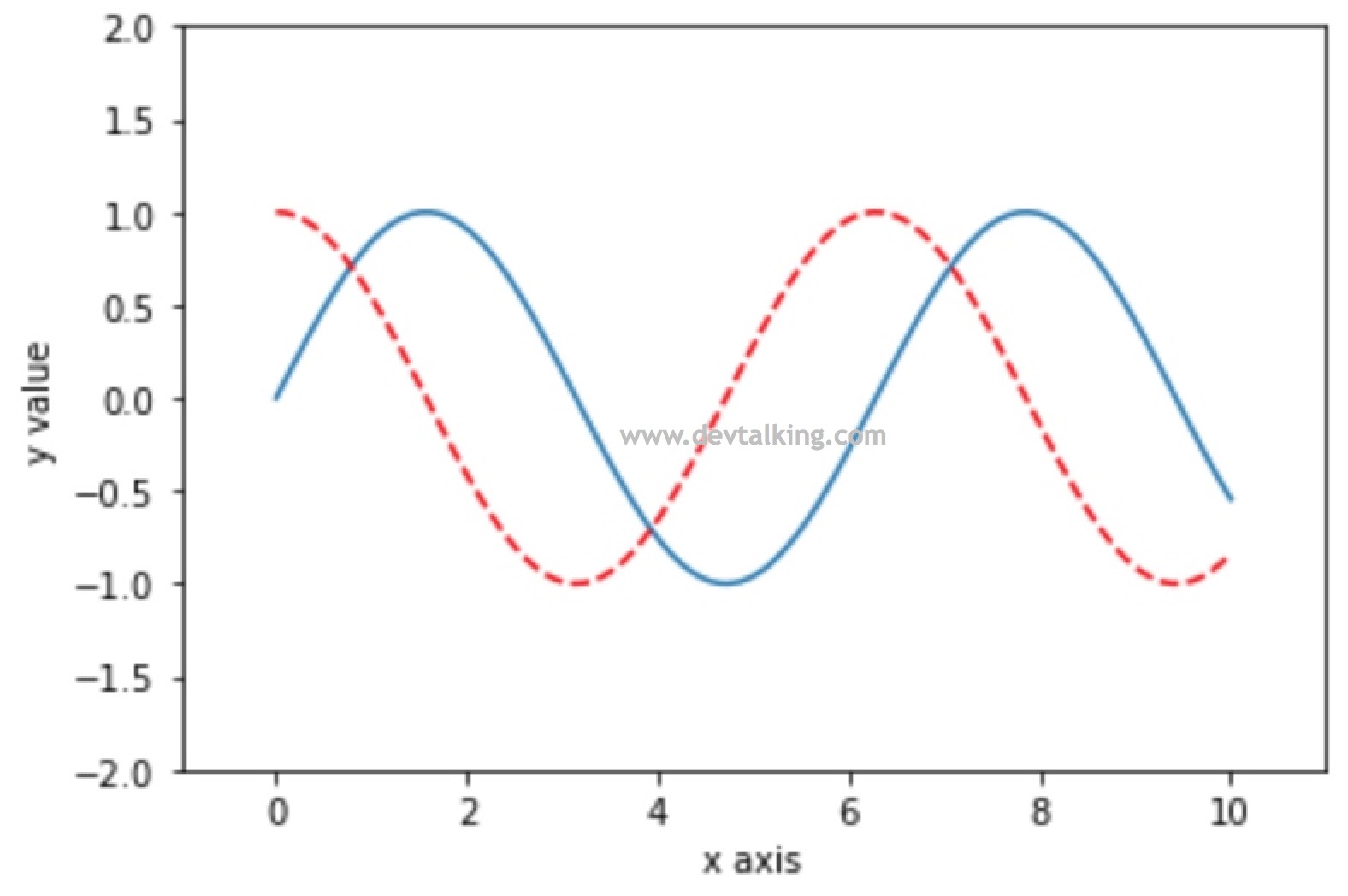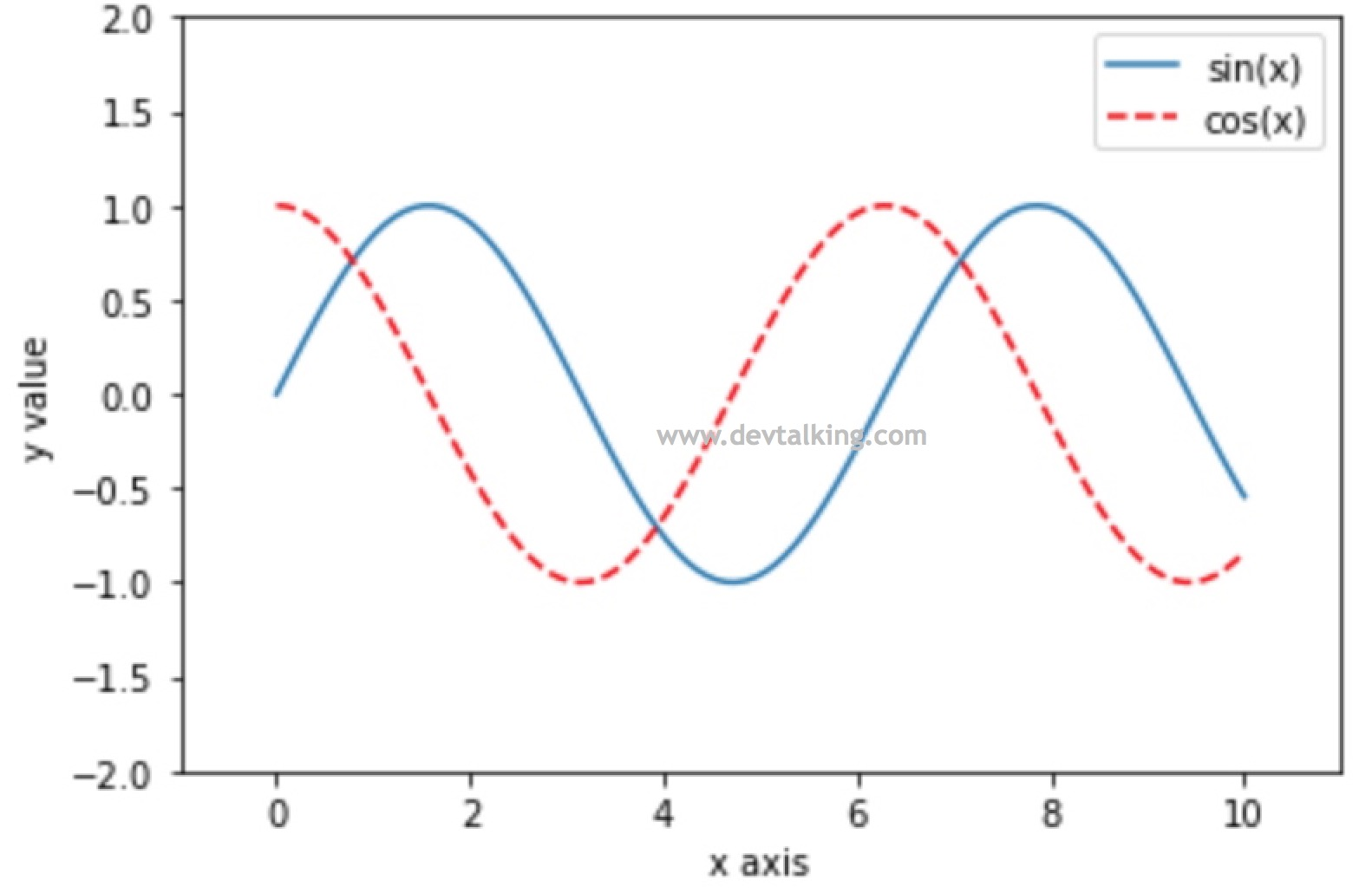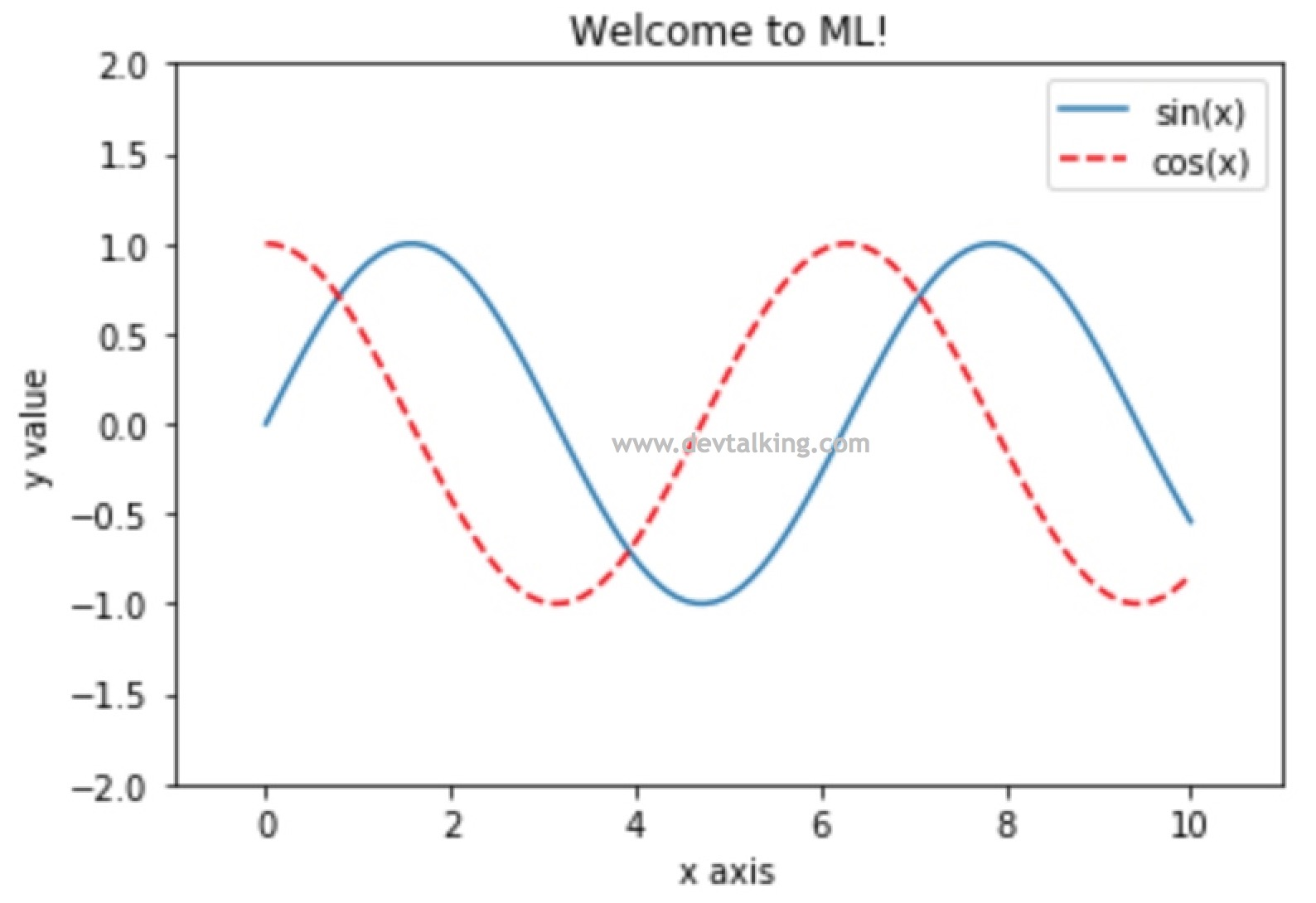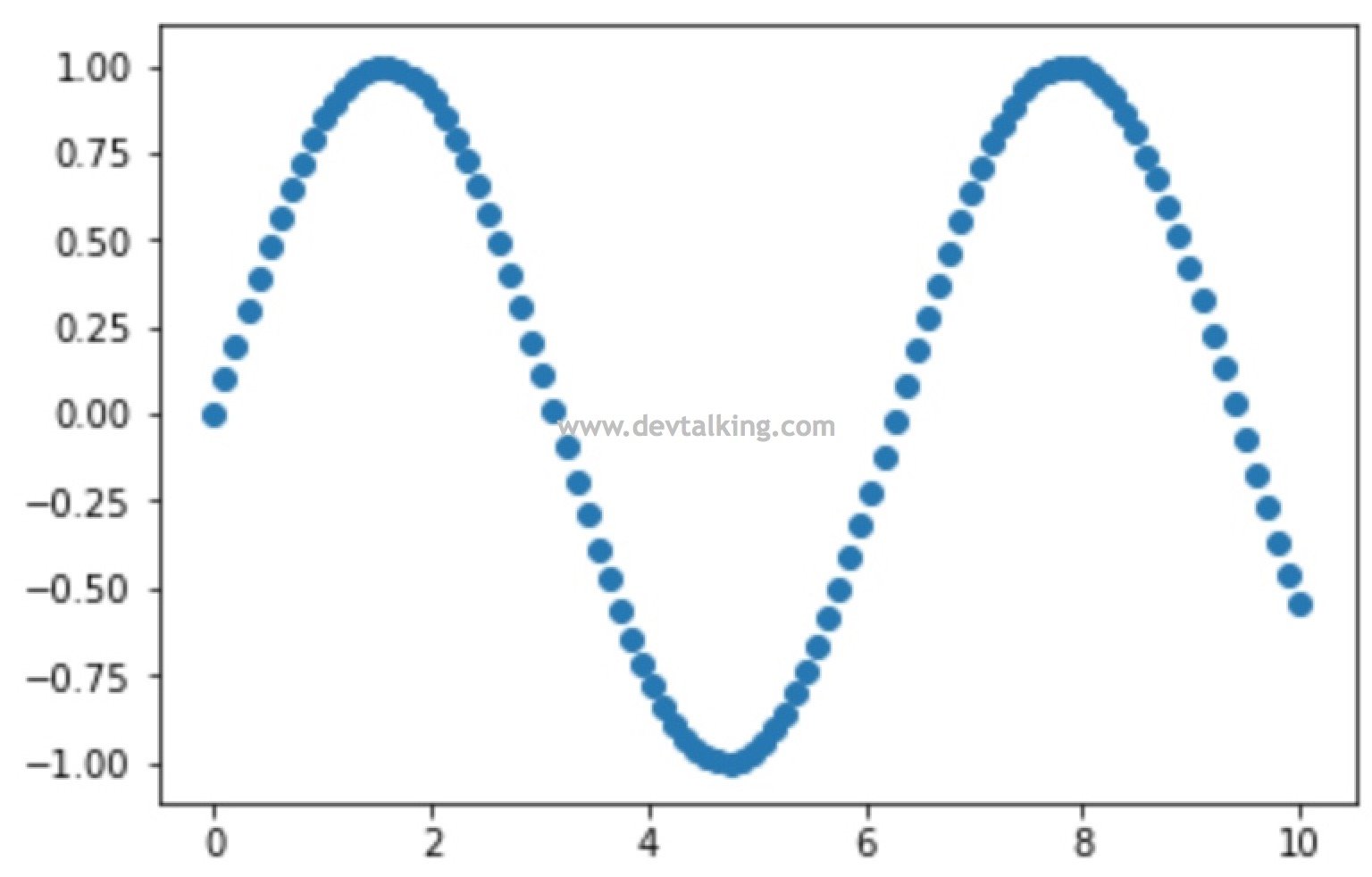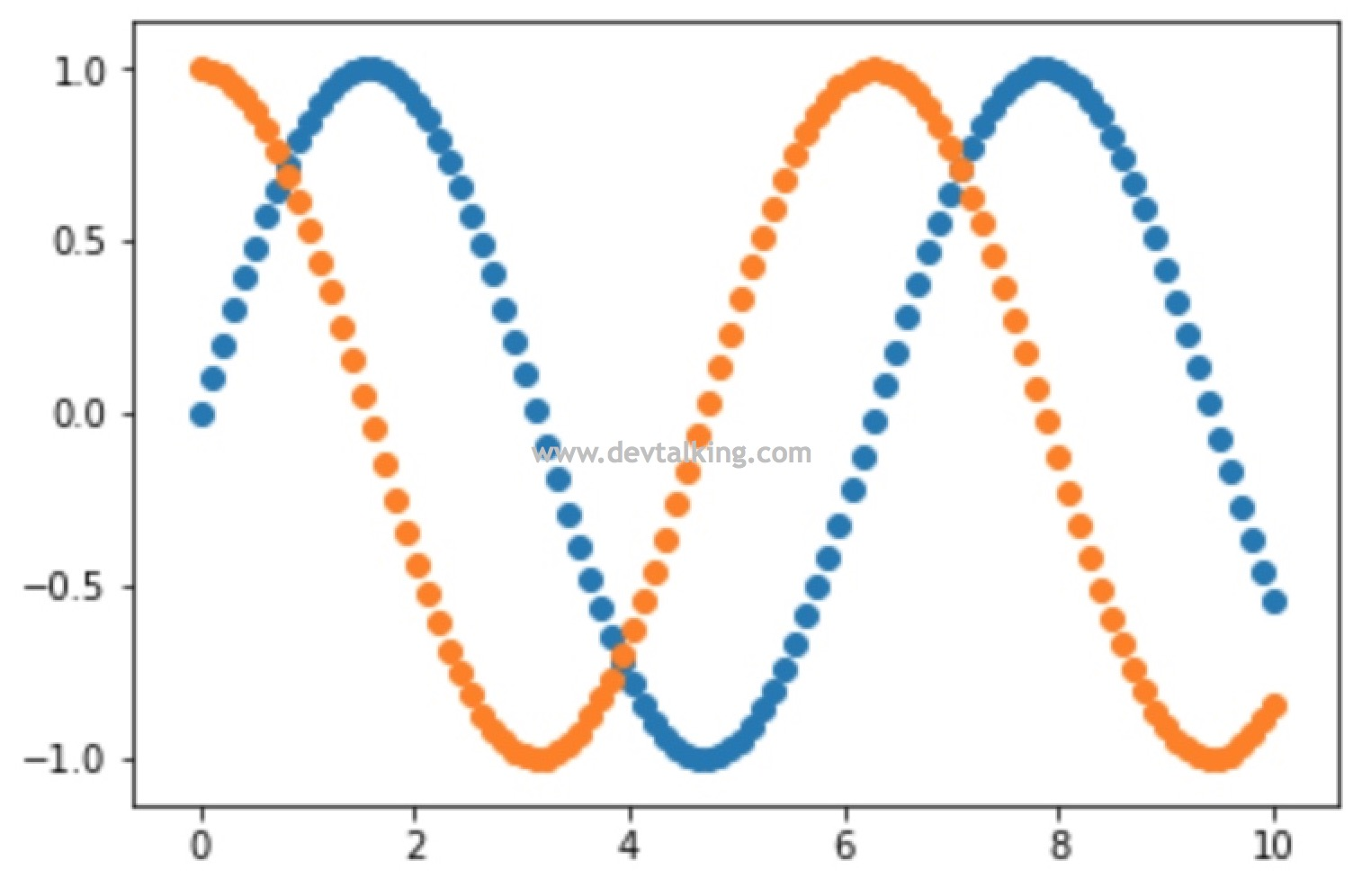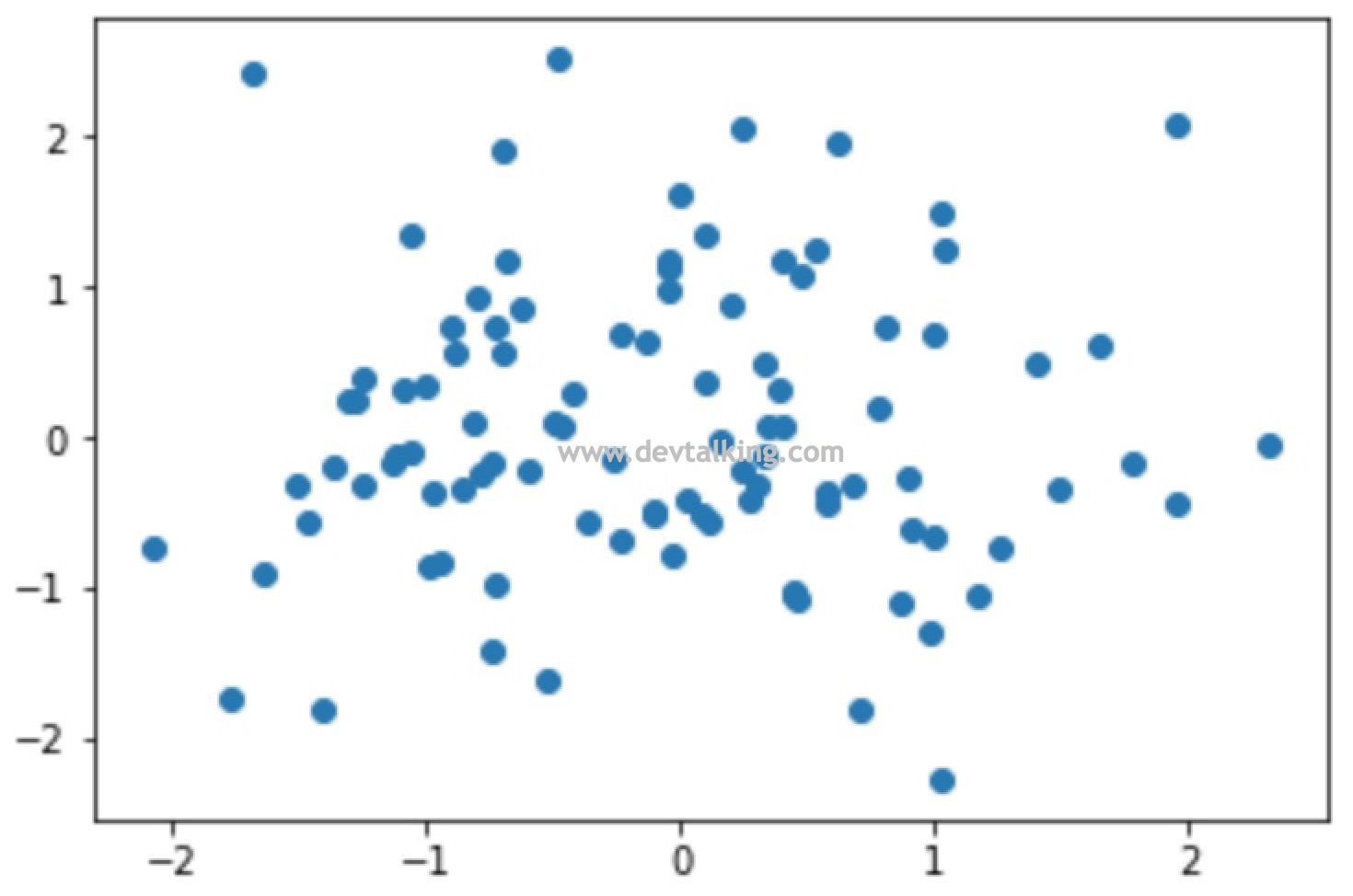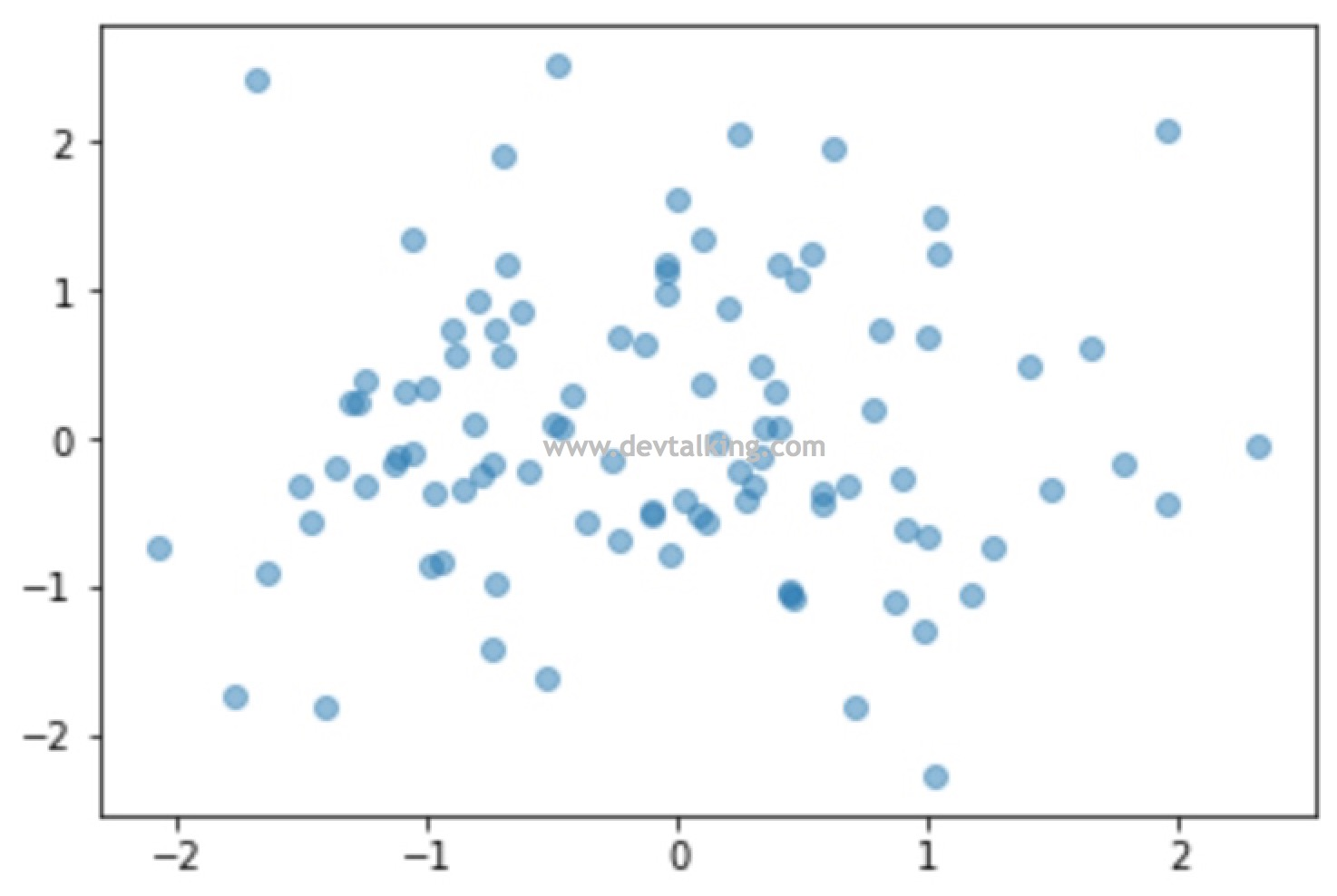基于Scikit Learn的数据探索

Scikit-learn是Python语言中专门针对机器学习应用而发展起来的一款开源框架，其中有一个模块叫Datasets，它提供了机器学习的一些常用的数据集以及产生数据集的方法，比如波士顿房价数据集、乳腺癌数据集、糖尿病数据集、手写字体数据集、鸢尾花数据集等等。这一小节我们就通过Scikit Learn的Datasets来初步对机器学习的数据进行探索。

DESCR详细的描述了鸢尾花这个数据集一共有150组数据，每组数据有4个特征，分别是萼片的长度和厚度、花瓣的长度和厚度，还有3种鸢尾花的类别以及这些数据的统计信息和详细的解释说明。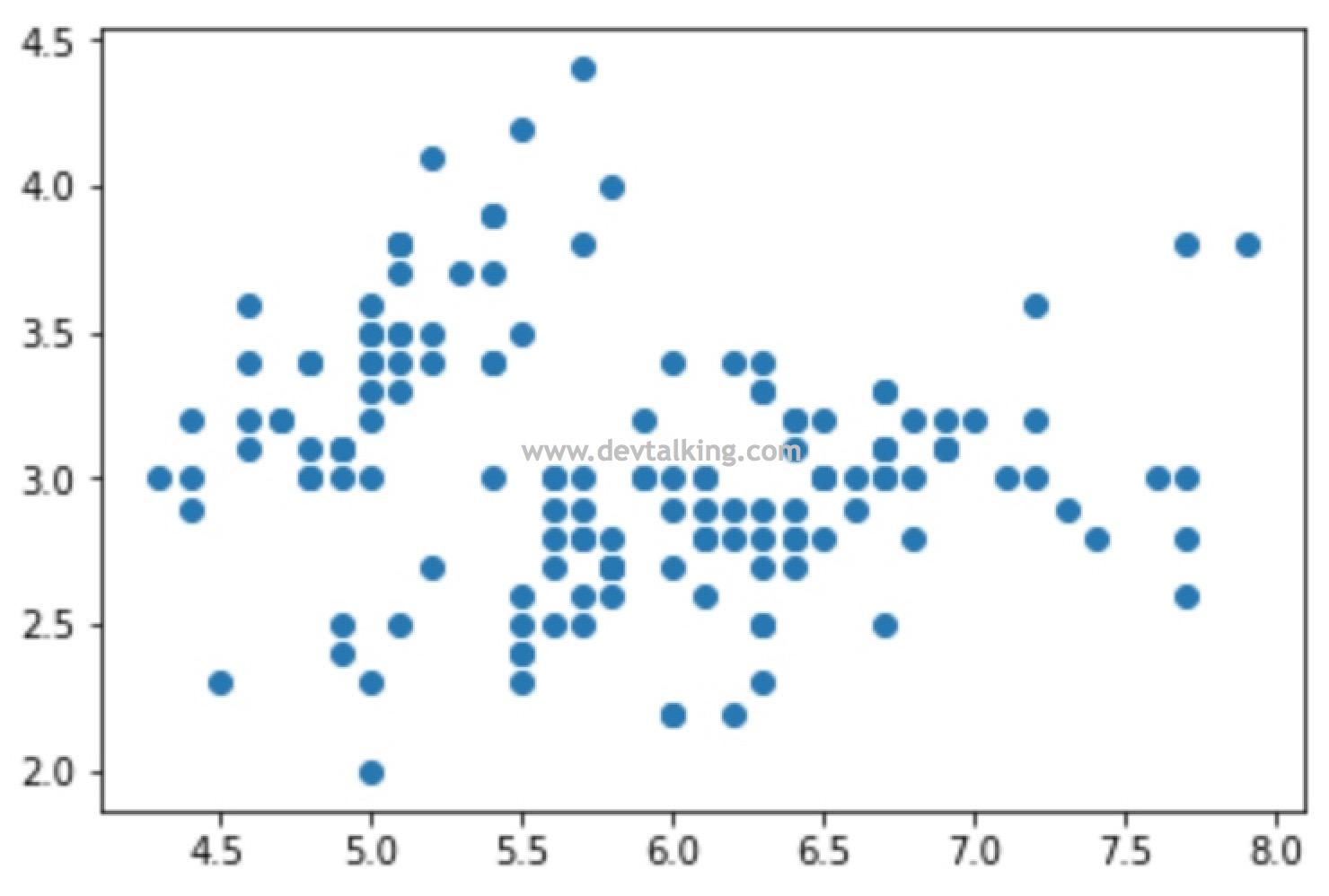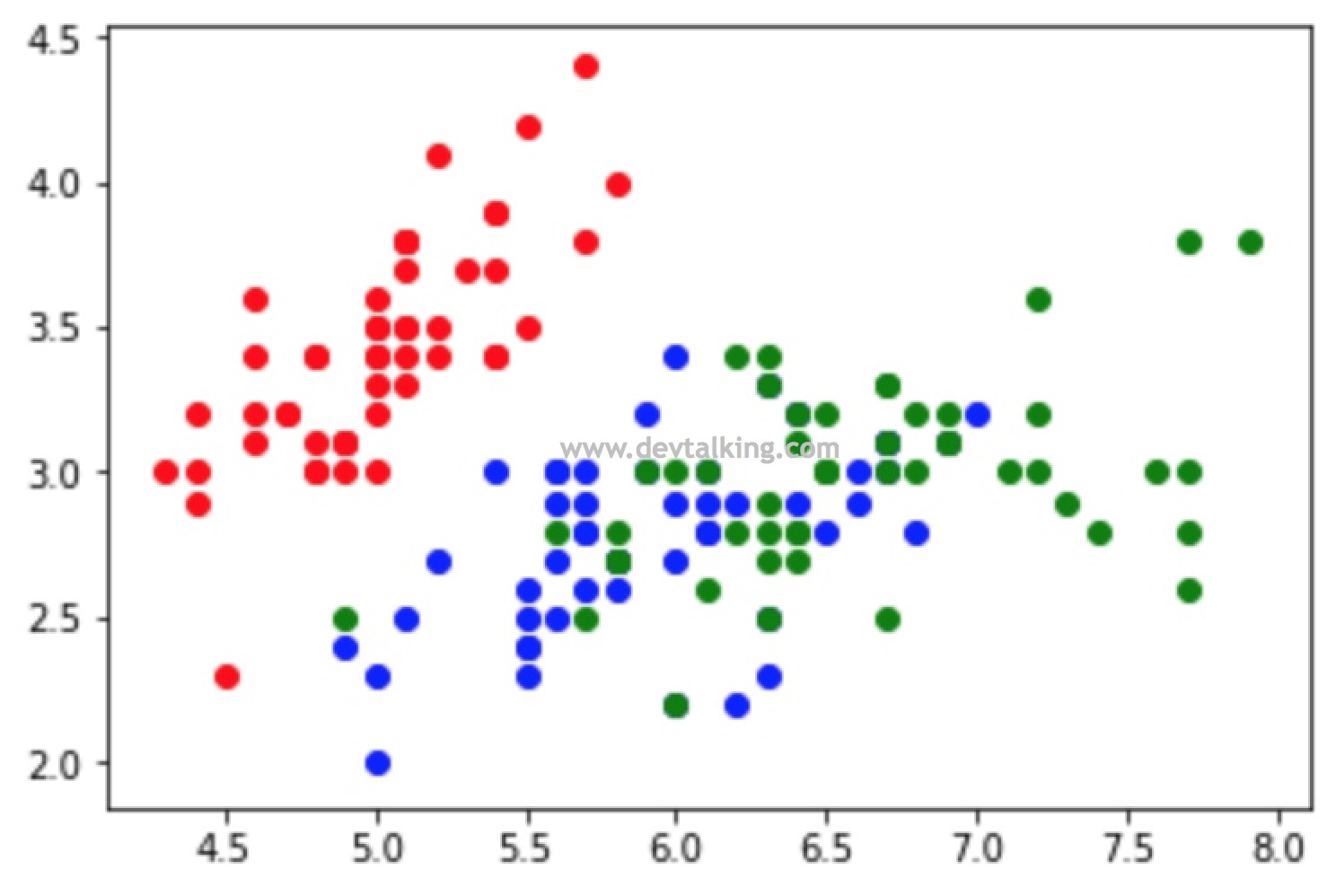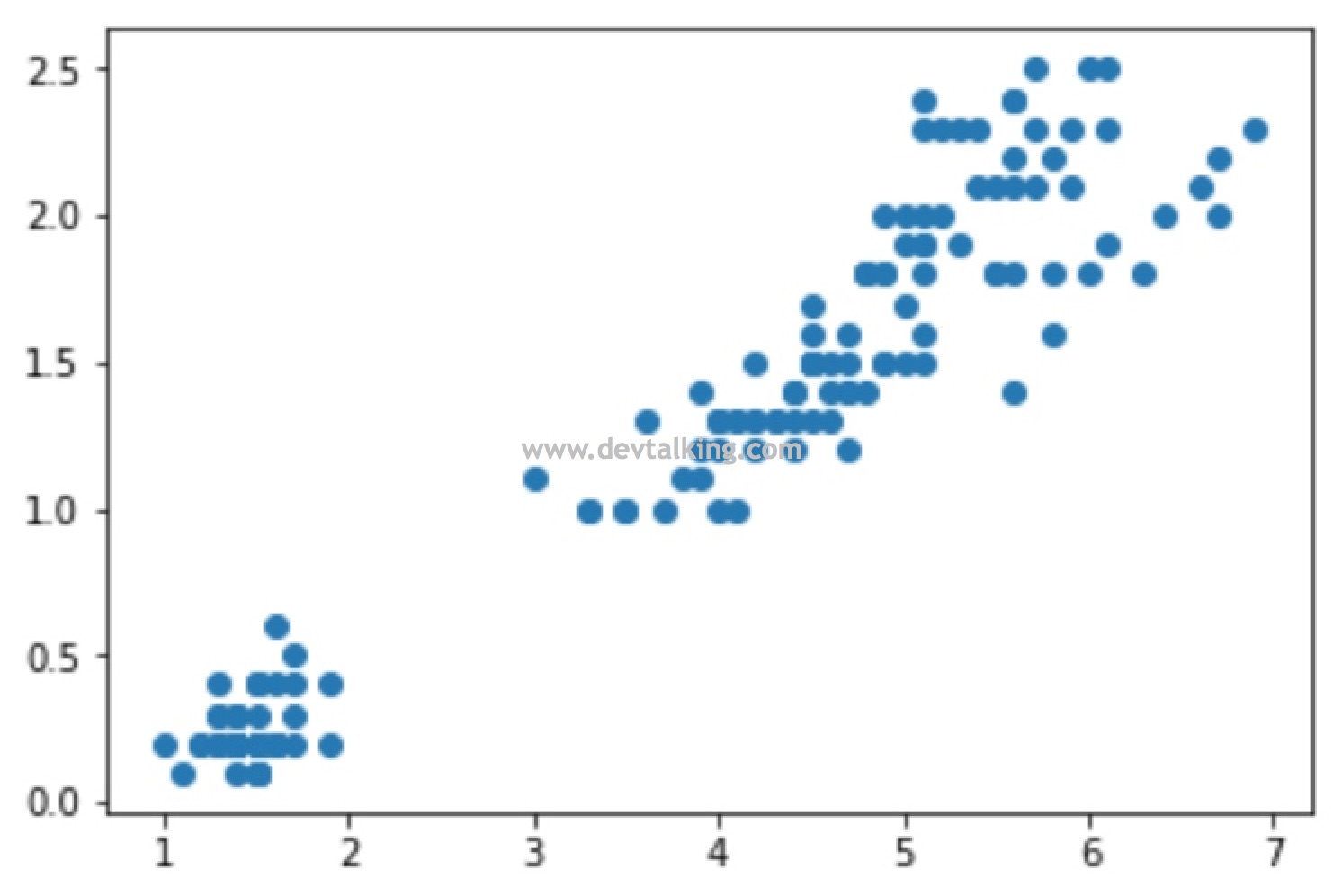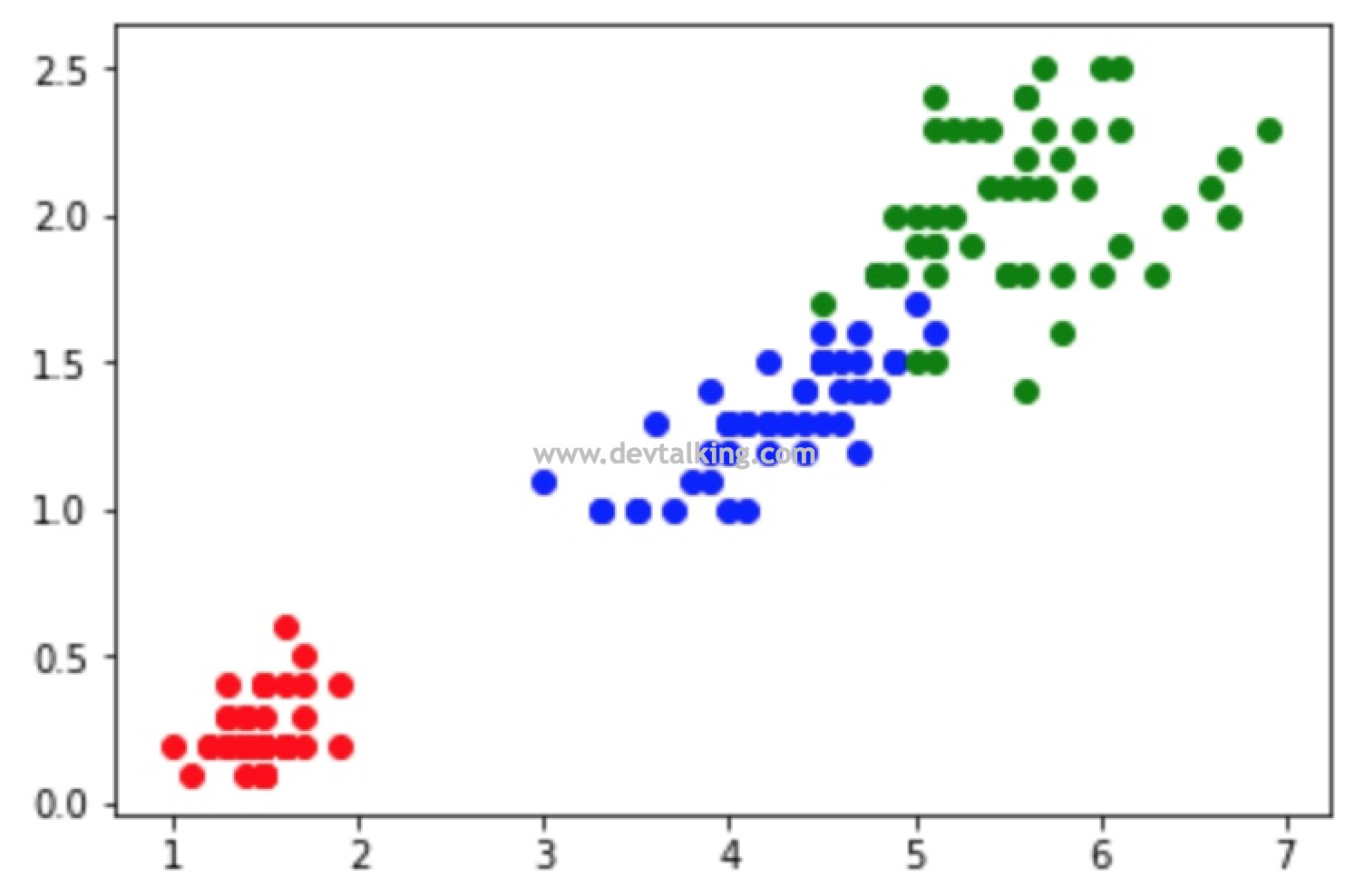kNN算法

kNN算法又称k近邻算法，是k-Nearest Neighbors的简称，该算法是监督学习中解决分类问题的算法，也是需要数据知识最少的一个算法，但是效果往往不差，能较好的解释机器学习算法使用过程中的很多细节问题，并且能很好的刻画机器学习应用的流程。

kNN算法解释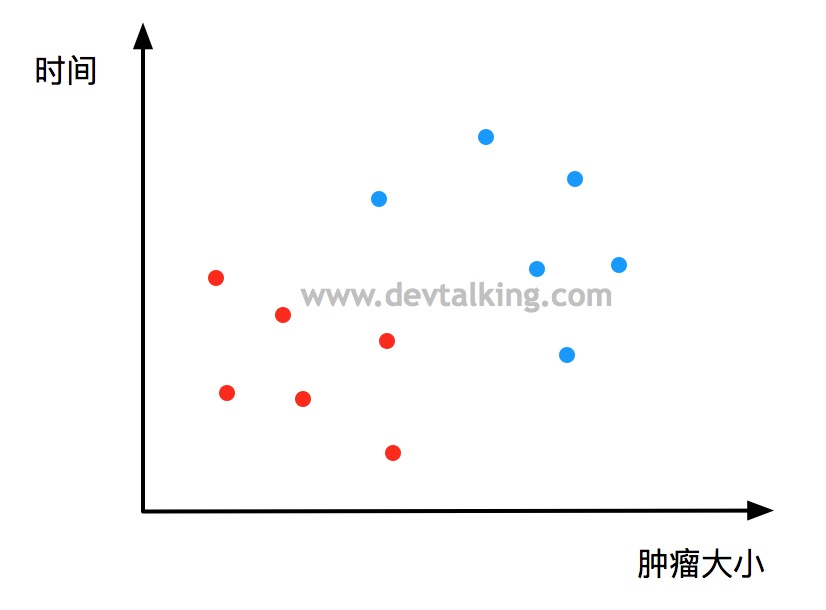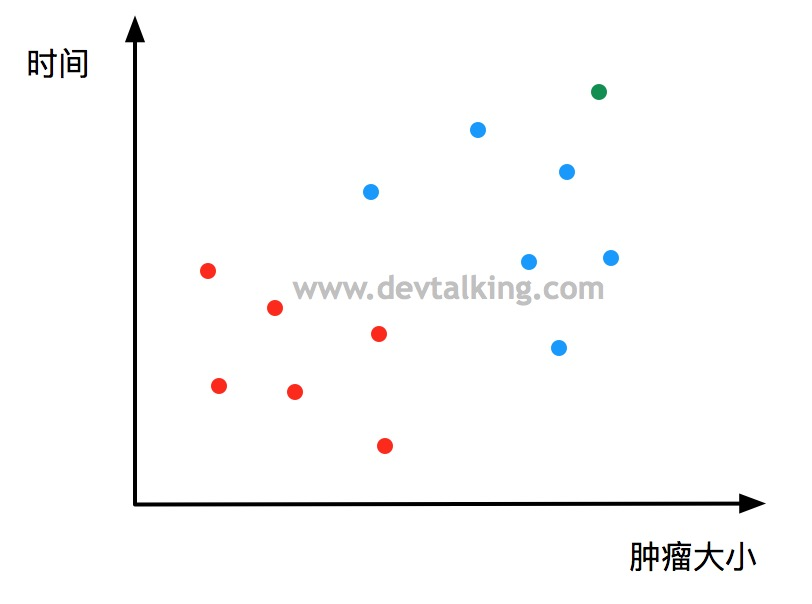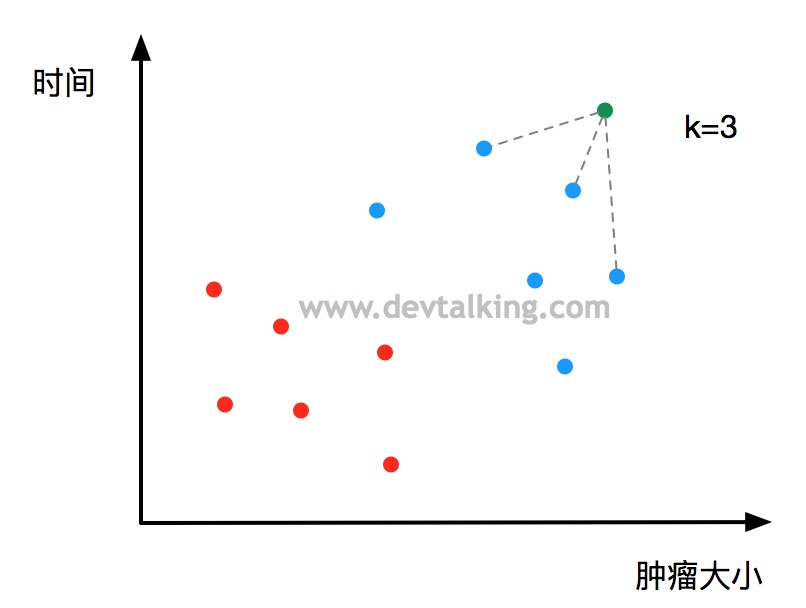欧拉距离

kNN算法中唯一用到的数学知识就是如何求点与点之间的距离，在这里我们先使用最普遍的欧拉距离来进行计算，欧拉距离的公式如下：

$$\sqrt {(x^{(a)}-x^{(b)})^2+(y^{(a)}-y^{(b)})^2}$$

$$\sqrt {(x^{(a)}-x^{(b)})^2+(y^{(a)}-y^{(b)})^2+(z^{(a)}-z^{(b)})^2}$$

N维(N个特征)：

$$\sqrt {(x_1^{(a)}-x_1^{(b)})^2+(x_2^{(a)}-x_2^{(b)})^2+…+(x_n^{(a)}-x_n^{(b)})^2} =\sqrt {\sum_{i=1}^n(x_i^{(a)}-x_i^{(b)})^2}$$

编码实现kNN算法